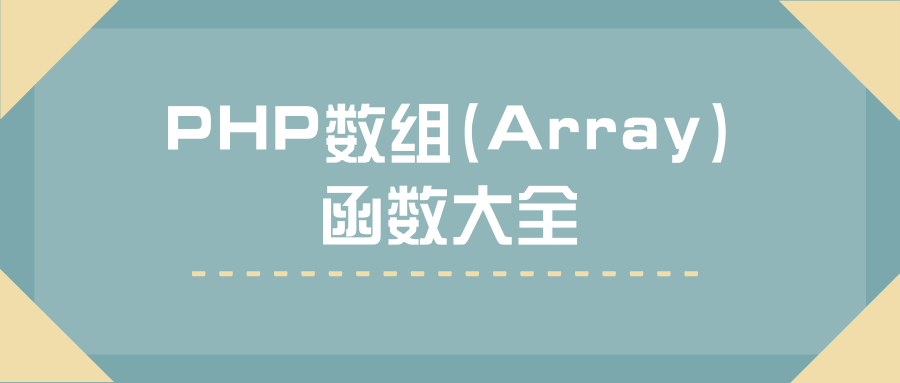# PHP开发中(Array)数组函数大全

5 篇文章 0 订阅### 一、数组基本操作函数

• 数组键值对操作
array_values($array); // 返回数组中所有的值 array_keys($array);     // 返回数组中所有的键名
array_flip($array); // 交换数组中的键和值 in_array($str,$array); // 检查数组中是否存在指定的值 array_search($str,$array); // 在数组中搜索某个键值，并返回对应的键名 array_key_exists($str,$array); // 检索给定的键名是否存在数组中  • 数组内部指针操作 current($array);     // 返回数组中的当前元素
pos($array); // current() 的别名，返回数组中的当前元素 key($array);      // 返回数组中当前元素的键名
prev($array); // 将数组的内部指针倒回一位 next($array);     // 将数组中的内部指针向前移动一位
end($array); // 将数组的内部指针指向最后一个元素 reset($array);    //  将数组的内部指针指向第一个元素
each($array); // 返回数组中当前的键／值对 list($key,$array) => each($array);    // 把数组中的值赋给一些变量

• 数组和变量之间的转换
extract($array); // 用于把数组中的元素转换成变量导入到当前文件中，键名当作变量名，值作为变量值 //注：（第二个参数很重要，可以看手册使用）使用方法 echo$a;
compact(var1,var2,var3);   // 用给定的变量名创建一个数组


### 二、数组的切割和填充

• 数组的分段
array_slice($array,0,3); // 返回数组中被选定的部分，例如：从第一个元素开始，截取3个长度 array_splice($array,0,3);  // 删除并替换数组中指定的元素，例如：从第一个元素开始，移除3个长度

• 分割多个数组
array_chunk($array,3,TRUE); // 可以将一个数组分割成多个，TRUE为保留原数组的键名  • 填充数组 array_pad($array,5,'x');    // 用值将数组填补到指定长度


### 三、数组与栈

array_push($array,"val1","val2"); // 将一个或多个元素压入数组栈的末尾（入栈），返回入栈元素的个数 array_pop($array);   // 将数组栈的最后一个元素弹出（出栈）


### 四、数组与队列

array_shift($array); // 数组中的第一个元素移出并作为结果返回（数组长度减1，其他元素向前移动一位，数字键名改为从零开始，文字键名不变） array_unshift($array,"a",array(1,2));   // 在数组的开头插入一个或多个元素


### 五、回调函数

array_walk($array,'function','words'); // 使用用户函数对数组中的每个成员进行处理（第三个参数传递给回调函数function） array_mpa("function",$array1,$array2); // 可以处理多个数组（当使用两个或更多数组时，他们的长度应该相同） array_filter($array,"function");       // 使用回调函数过滤数组中的每个元素，如果回调函数为TRUE，数组的当前元素会被包含在返回的结果数组中，数组的键名保留不变
array_reduce($array,"function","*"); //转化为单值函数（*为数组的第一个值  ### 六、数组排序 • 通过元素排序 sort($array);    // 由小到大的顺序排序（第二个参数为按什么方式排序）忽略键名的数组排序
rsort($array); // 由大到小的顺序排序（第二个参数为按什么方式排序）忽略键名的数组排序 usort($array,"function");   // 使用用户自定义的比较函数对数组中的值进行排序（function中有两个参数，0表示相等，正数表示第一个大于第二个，负数表示第一个小于第二个）忽略键名的数组排序
asort($array); // 由小到大的顺序排序（第二个参数为按什么方式排序）保留键名的数组排序 arsort($array);  // 由大到小的顺序排序（第二个参数为按什么方式排序）保留键名的数组排序
uasort($array,"function"); // 使用用户自定义的比较函数对数组中的值进行排序（function中有两个参数，0表示相等，正数表示第一个大于第二个，负数表示第一个小于第二个）保留键名的数组排  • 通过键名排序 ksort($array);   // 按照键名正序排序
krsort($array); // 按照键名逆序排序 uksort($array,"function");   // 使用用户自定义的比较函数对数组中的键名进行排序（function中有两个参数，0表示相等，正数表示第一个大于第二个，负数表示第一个小于第二个）

• 自然排序
// 在自然排序算法中，数字 2 小于 数字 10。在计算机排序算法中，10 小于 2，因为 "10" 中的第一个数字小于 2
// 即数字从 1 到 9 的排序方法，字母从 a 到 z 的排序方法，短者优先。数组的索引与单元值保持关联。
natsort($array); // 自然排序（忽略键名） natcasesort($array);   // 自然排序，该函数对大小写不敏感（忽略大小写，忽略键名）


### 七、数组的运算

• 数组元素求和
array_sum($array); // 对数组内部的所有元素做求和运算  • 数组合并 // 合并两个或多个数组（相同的字符串键名，后面的覆盖前面的，相同的数字键名，后面的不会做覆盖操作，而是附加到后面） // “+”$array1+$array2; 对于相同的键名只保留后一个 array_merge($array1,$array2); // 递归合并操作，如果数组中有相同的字符串键名，这些值将被合并到一个数组中去。 // 如果一个值本身是一个数组，将按照相应的键名把它合并为另一个数组。 // 当数组 具有相同的数组键名时，后一个值将不会覆盖原来的值，而是附加到后面 array_merge_recursive($array1,$array2);  • 数组差集 array_diff($array1,$array2); // 返回差集结果数组 array_diff_assoc($array1,$array2,$array3);   // 返回差集结果数组，键名也做比较

• 数组交集
array_intersect($array1,$array2);    // 比较数组，返回交集（只比较键值）
array_intersect_assoc($array1,$array2);   // 比较数组，返回交集（比较键名和键值，使用用户自定义的键名比较函数）
array_intersect_key($array1,$array2);    // 比较数组，返回交集（只比较键名）
array_intersect_ukey($array1,$array2);  // 比较数组，返回交集（只比较键名，使用用户自定义的键名比较函数）


### 八、其他数组函数

• 本类函数允许用多种方法来操作数组和与之交互。数组的本质是储存，管理和操作一组变量。PHP 支持一维和多维数组，可以是用户创建或由另一个函数创建。有一些特定的数据库处理函数可以从数据库查询中生成数组，还有一些函数返回数组。
range(0,12);    // 创建一个包含指定范围单元的数组
array_unique($array); // 移除数组中重复的值，新的数组中会保留原始的键名 array_reverse($array,TRUE);  //返回一个单元顺序与原数组相反的数组，如果第二个参数为TRUE保留原来的键名
//srand((float)microtime()*10000000);   // 随机种子触发器
array_rand($array,2); // 从数组中随机取出一个或 多个元素 shuffle($array);   // 将数组的顺序打乱
array_change_key_case($array,CASE_LOWER); // 返回字符串键名全为小写或大写的数组，可选。可能的值：CASE_LOWER - (默认值)将数组的键转换为小写字母。CASE_UPPER - 将数组的键转换为大写字母。 array_combine($keyArr,$valArr); // 创建一个数组，用一个数组的值作为其键名，另一个数组的值作为其值 array_count_values($arr);  // 统计数组中所有的值出现的次数
array_fill_keys($array,"key1"); //用指定键名的给定键值填充数组 array_fill($index,\$number,"key1");  // 用给定的值填充数组，index - 规定要插入的元素数，number - 规定要插入的元素数


array_filter — 用回调函数过滤数组中的单元
array_flip — 交换数组中的键和值
array_key_exists — 检查给定的键名或索引是否存在于数组中
array_keys — 返回数组中所有的键名
array_map — 将回调函数作用到给定数组的单元上
array_multisort — 对多个数组或多维数组进行排序
array_product — 计算数组中所有值的乘积
array_rand — 从数组中随机取出一个或多个单元
array_reduce — 用回调函数迭代地将数组简化为单一的值
array_reverse — 返回一个单元顺序相反的数组
array_search — 在数组中搜索给定的值，如果成功则返回相应的键名
array_shift — 将数组开头的单元移出数组
array_unique — 移除数组中重复的值
array_unshift — 在数组开头插入一个或多个单元
array_walk_recursive — 对数组中的每个成员递归地应用用户函数
array_walk — 对数组中的每个成员应用用户函数
array — 新建一个数组
compact — 建立一个数组，包括变量名和它们的值
count — 计算数组中的单元数目或对象中的属性个数
range — 建立一个包含指定范围单元的数组
shuffle — 将数组打乱
sizeof — count() 的别名10-20
05-111万+
11-154万+
12-01570
12-0362
12-01528
11-29294
11-29547点击重新获取扫码支付余额充值